# Angle Bisector

Angle bisector in geometry refers to a line that splits an angle into two equal angles. Bisector means the thing that bisects a shape or an object into two equal parts.  If we draw a ray that bisects an angle into two equal parts of the same measure, then it is called an angle bisector.

Before talking about an angle bisector, let us quickly recall the different types of angles in mathematics. Depending on the inclination between the two arms, an angle may be acute (less than 90-degrees, like 60-degree angle), obtuse (more than 90-degrees) or right angle (exactly 90-degrees). Constructing angles is an important part of geometry as this knowledge is extended for the construction of other geometric figures as well, primarily the triangles. A number of angles can be constructed simply by bisecting some common angles.

## What is an Angle Bisector?

An angle bisector or the bisector of an angle is a ray that divides an angle into two equal parts. For example, if a ray KM divides an angle of 60 degrees into two equal parts, then each measure will be equal to 30 degrees.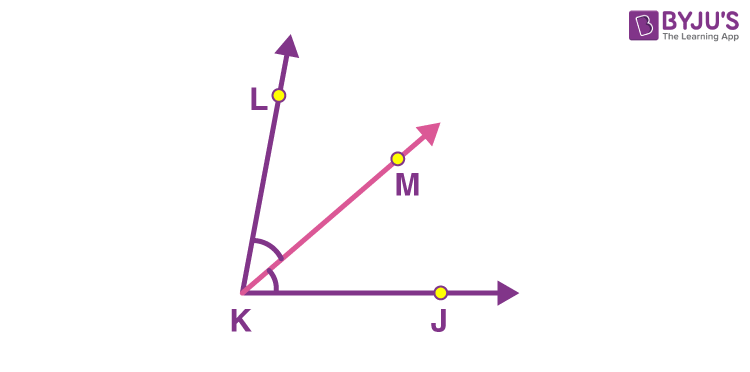Every angle has an angle bisector. It is also the line of symmetry between the two arms of an angle, the construction of which enables you to construct smaller angles. Say you are required to construct a 30° angle. This can be performed by creating a 60° angle and then bisecting it. Similarly, 90-degree, 45-degree, 15-degree and other angles are constructed using the concept of an angle bisector.

## Properties of Angle Bisector

• All the points of angle bisector are equidistant from both the arms of the angle
• An angle bisector can be drawn to any angle, such as acute, obtuse, or right angle
• The angle bisector in a triangle divides the opposite side in a ratio that is equal to the ratio of the other two sides

## How to Construct an Angle Bisector?

You require a ruler and a compass to construct angles and their bisectors. Given a known or unknown ∠PQR, the steps to construct its angle bisector are: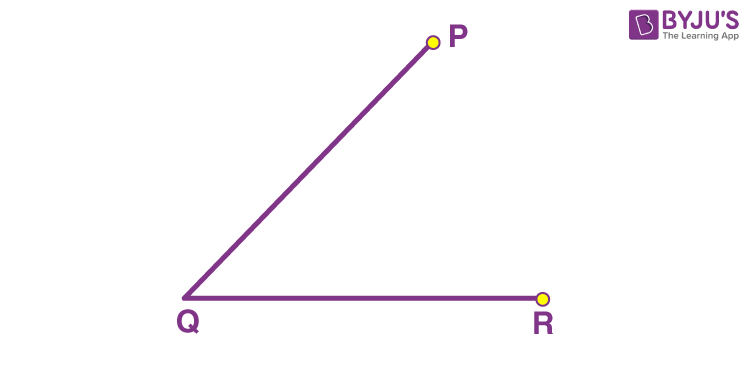Step 1: Place the compass pointer at Q and make an arc that cuts the two arms of the angle at two different points.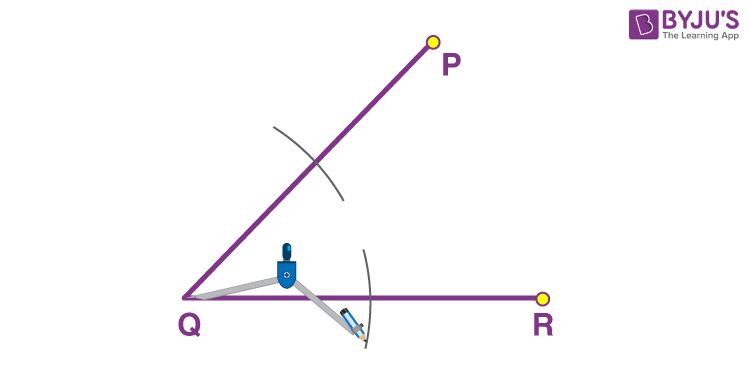Step 2: From the point where the first arc cut the arm QP, make another arc towards the interior of the angle.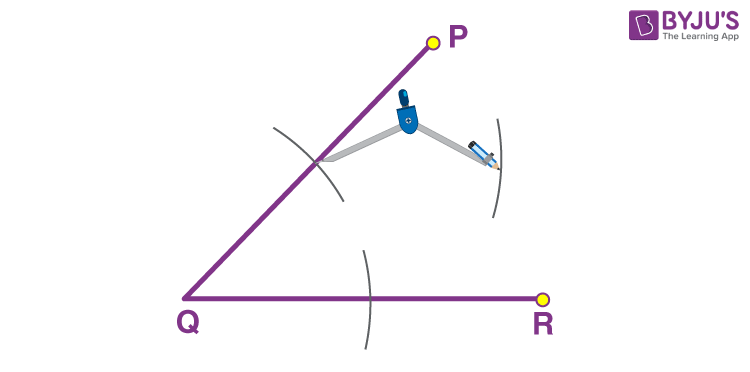Step 3: Without changing the radius on the compass, repeat step 2 from the point where the first arc cut QR.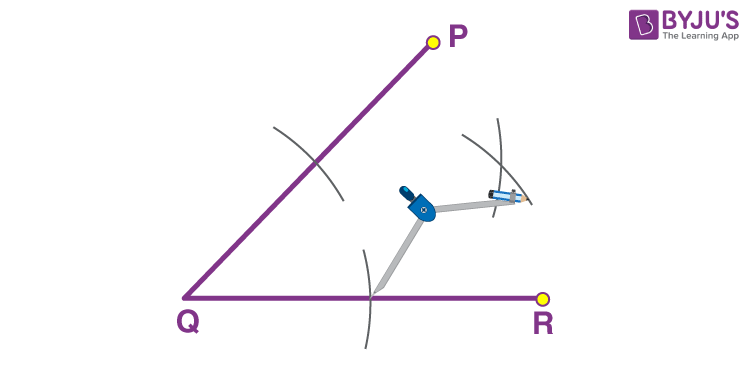Step 4: Using a ruler, draw a line from Q to the point where the arcs intersect.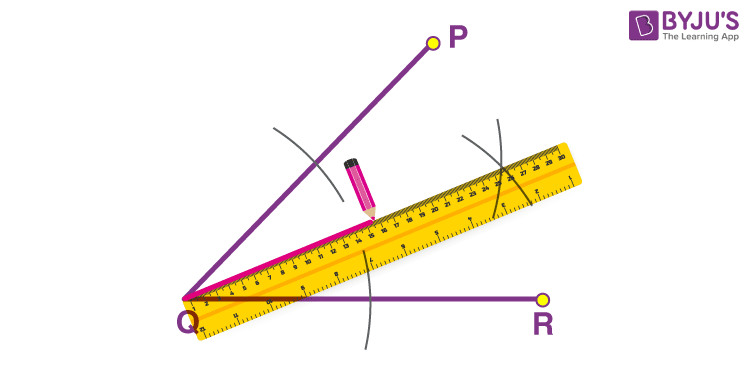The line that was drawn through Q represents the angle bisector of the ∠PQR.

Note: If an angle bisector bisects a line segment at 90°, it is known as the perpendicular bisector of that line.

## Video Lesson on Angle Bisector## Angle Bisector Theorem

According to the angle bisector theorem, “an angle bisector of a triangle divides the opposite side into two segments that are proportional to the other two sides of the triangle”.

## Solved Examples on Angle Bisector

Q.1: If an angle bisector divides an angle of 80 degrees, then what is the measure of each angle?

Solution: Given, a measure of an angle is 80 degrees

As we know, the angle bisector divides the angle into equal two parts.

Therefore, 80 degrees is divided into equal two parts, say x.

Hence,

x + x = 80°

2x = 80°

x = 80°/2

x = 40°

Q.2: A ray BX, divides an angle ABC into two equal parts. If one part is equal to 4x – 5 and the second part is equal to 20, then what is the value of x?

Solution: Given, BX divides angle ABC into two equal parts. Thus, BX is the angle bisector.

Now, each part should measure equal.

Thus,

4x – 8 = 20

4x = 20 + 8 = 28

x = 28/4 = 7

Hence, the value of x is 7.

## Practice Questions on Angle Bisector

1. If an angle bisector divides 90 degrees, then what is the measure of each angle?
2. Construct the angle bisector of 110 degrees angle.
3. Construct an angle bisector of 30 degrees angle.

## Frequently Asked Questions on Angle bisector

Q1

### What do you mean by angle bisector?

An angle bisector is a ray that divides an angle into two parts of equal measure.
Q2

### What are the properties of angle bisectors?

The properties of angle bisector are:
All the points of angle bisector are equidistant from both the arms of the angle
An angle bisector can be drawn to any angle, such as acute, obtuse, or right angle
The angle bisector in a triangle divides the opposite side in a ratio that is equal to the ratio of the other two sides
Q3

### Does the angle bisector of a triangle divide the sides?

The angle bisector of a triangle divides the opposite sides in a ratio proportional to the other two sides of the triangle.
Q4

### How many angle bisectors does an angle have?

There is only an angle bisector to an angle.